# vs.eyeandcontacts.com

## Chapter 7 Congruence of Triangles Exercise 7.2

Question 1: Which congruence criterion do you use in the following?
(a) Given: AC = DF
AB = DE
BC = EF
So, ΔABC ≅ ΔDEF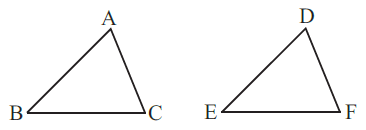∆ABC ≅ ∆DEF (by SSS rule)

(b) Given: ZX = RP
RQ = ZY
∠PRQ = ∠XZY
So, ΔPQR ≅ ΔXYZ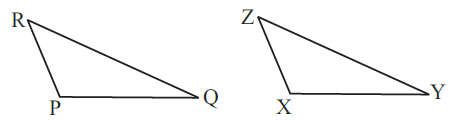∆PQR ≅ ∆XYZ (BY SAS rule)

(c) Given: ∠MLN = ∠FGH
∠NML = ∠GFH
∠ML = ∠FG
So, ΔLMN ≅ ΔGFH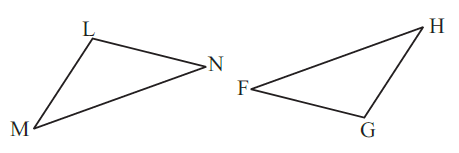∆LMN ≅ ∆GFH (BY ASA rule)

(d) Given: EB = DB
AE = BC
∠A = ∠C = 90°
So, ΔABE ≅ ΔACD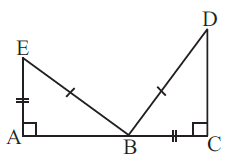∆ABE ≅ ∆CDB (BY RHS rule)

Question 2: You want to show that ΔART ≅ ΔPEN,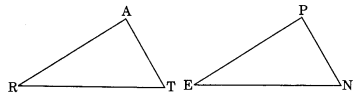(a) If you have to use SSS criterion, then you need to show

(i) AR = PE
(ii) RT =
EN
(iii) AT =
PN

(b) If it is given that ∠T = ∠N and you are to use SAS criterion, you need to have
(i) RT = EN
(ii) PN =
AT

(c) If it is given that AT = PN and you are to use ASA criterion, you need to have
(i) and (ii)

(i) ∠ATR = ∠PNE
(ii) ∠RAT =
∠EPN

Question 3: You have to show that ΔAMP ≅ ΔAMQ.
In the following proof, supply the missing reasons.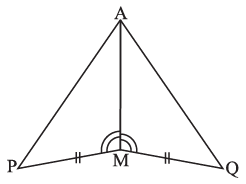Steps Reasons (i) PM = QM (i) … (ii) ∠PMA = ∠QMA (ii) … (iii) AM = AM (iii) … (iv) ΔAMP ≅ ΔAMQ (iv) …

 Steps Reasons (i) PM = QM from the given figure (ii) ∠PMA = ∠QMA from the given figure (iii) AM = AM common side for the both triangles (iv) ΔAMP ≅ ΔAMQ (by SAS congruence property) Two triangles are congruent if the two sides and the included angle of one are equal to the two sides and the included angle of the other.

Question 4: In ΔABC, ∠A = 30°, ∠B = 40° and ∠C = 110°
In ΔPQR, ∠P = 30°, ∠Q = 40° and ∠R = 110°
A student says that ΔABC ≅ ΔPQR by AAA congruence criterion. Is he justified? Why or Why not?
The student is not justified because AAA congruence criterion do not exist.

Question 5: In the figure, the two triangles are congruent. The corresponding parts are marked. We can write ΔRAT ≅ ?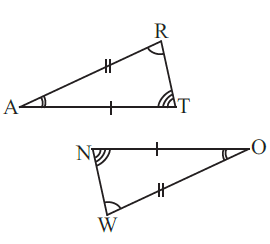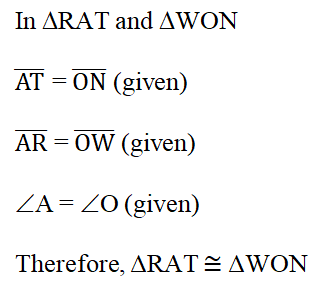Question 6: Complete the congruence statement: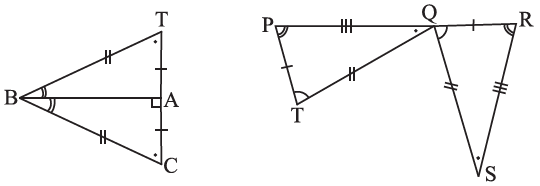ΔBCA ≅ ΔQRS ≅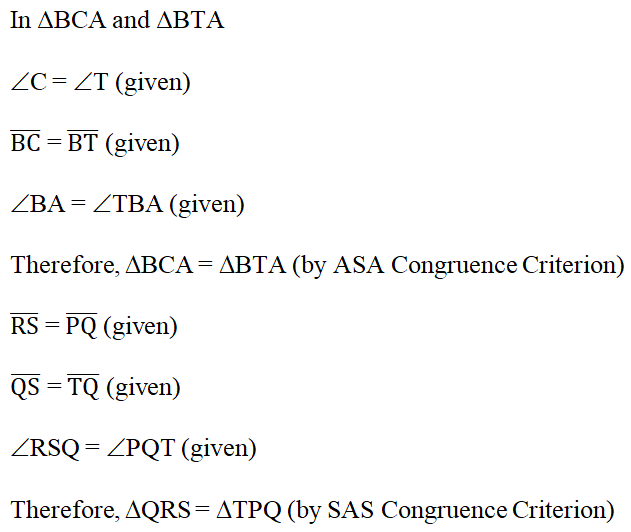Question 7: In a squared sheet, draw two triangles of equal areas such that
(i) The triangles are congruent.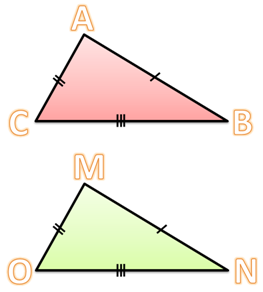⃤  ABC and  ⃤  MNO have the same length. So the area of these triangles is the same.

(ii) The triangles are not congruent.
What can you say about their perimeters?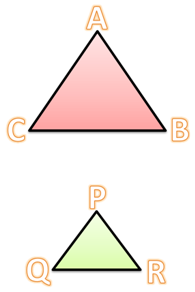⃤  ABC and  ⃤  PQR do not have the same length. So the area of these triangles is not the same.

Question 8: Draw a rough sketch of two triangles such that they have five pairs of congruent parts but still the triangles are not congruent.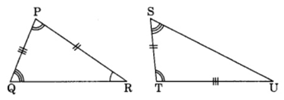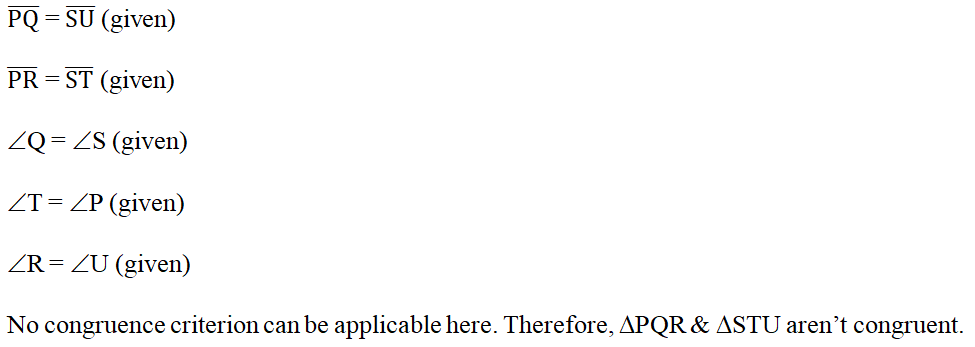Question 9: If ΔABC and ΔPQR are to be congruent, name one additional pair of corresponding parts. What criterion did you use?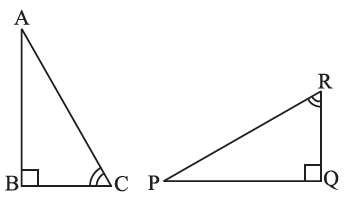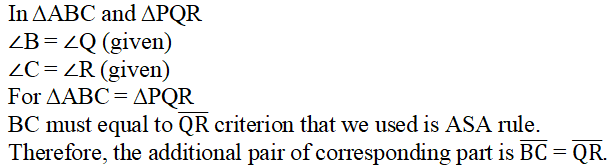Question 10: Explain, why ΔABC ≅ ΔFED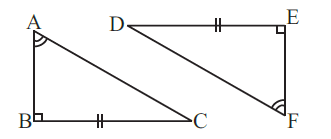In ∆ABC and ∆FED
∠B = ∠E = 90° (given)
∠A = ∠F (given)
Therefore, ∠A + ∠B = ∠E + ∠F
180° – ∠C = 180° – ∠D

(by Angle sum property)
Therefore, ∠C =∠D
BC = ED (given)
Therefore, ∆ABC = ∆FED (by ASA Congruence Criterion)